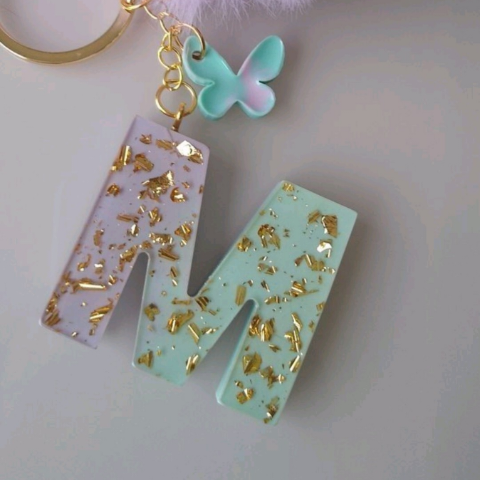# What will come at the place of question mark ?1, 9, 25, 49, ?, 121.

A. 100

B. 91

C. 64

D. 81

### Solution(By Examveda Team)

12 = 1.
32 = 9.
52 = 25.
72 = 49.
92 = 81.
112 = 121.

1.hello

2.1+8=9
9+16=25
25+24=49
49+32=81
81+40=121

So 81 is the answr

3.9-1=8=8x1
25-9=16=8x2
49-25=24=8x3
?-49=32=8x4
next series will be 49+32=81

Related Questions on Number Series Completion